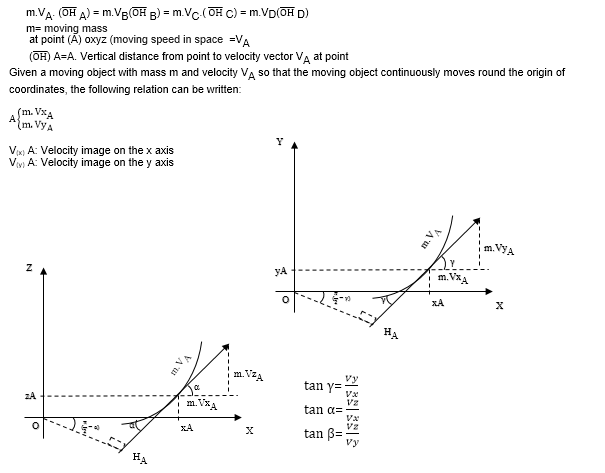Select Page

# Stable Dynamics (5th dimension)

Dynamics features movement and stable means

#### Physics , Mechanic , Mathematic

Dynamics features movement and stable means. Continuous

Stable dynamics thus means continuous movement or motion. That is a moving object which enjoys continuous movement. For example, the electron continuous revolution round the nucleus, the revolution of the moon round the earth and that of the earth round the sun. In this formula, the continuous movement of the moving object round the origin of coordinates in space is studied.

Regarding the importance of masses movement in space, the necessity is felt that in order to design and optimize dynamic systems (dynamic mechanics) and all relevant subsets, a reasonable relation should be presented (Some scientists believe that a charged particle is the 5th dimension in which case a particle enjoying continuous movement can be named the 5th dimension.

Key words: stable dynamics, materials

Introduction

In dynamic mechanics literature, the issue of movement relevant to a moving object is discussed and proved.

As an example, the throwing of a particle in space, a moving object range, force driving apogee, mass and acceleration of the moving object are discussed and have documented and compiled formulas.

In this formula, a moving object continuous movement is studied, and as detailed in the abstract, the formula of the moving object continuous movement, that is, the same stable dynamics, has been established.

The formula in question is initially proved in plane (xoy) and is then extended to planes (xoz) and (yoz).

In the end, we have 3 images of the moving object in the above mentioned planes to obtain the moving object general formula in space.